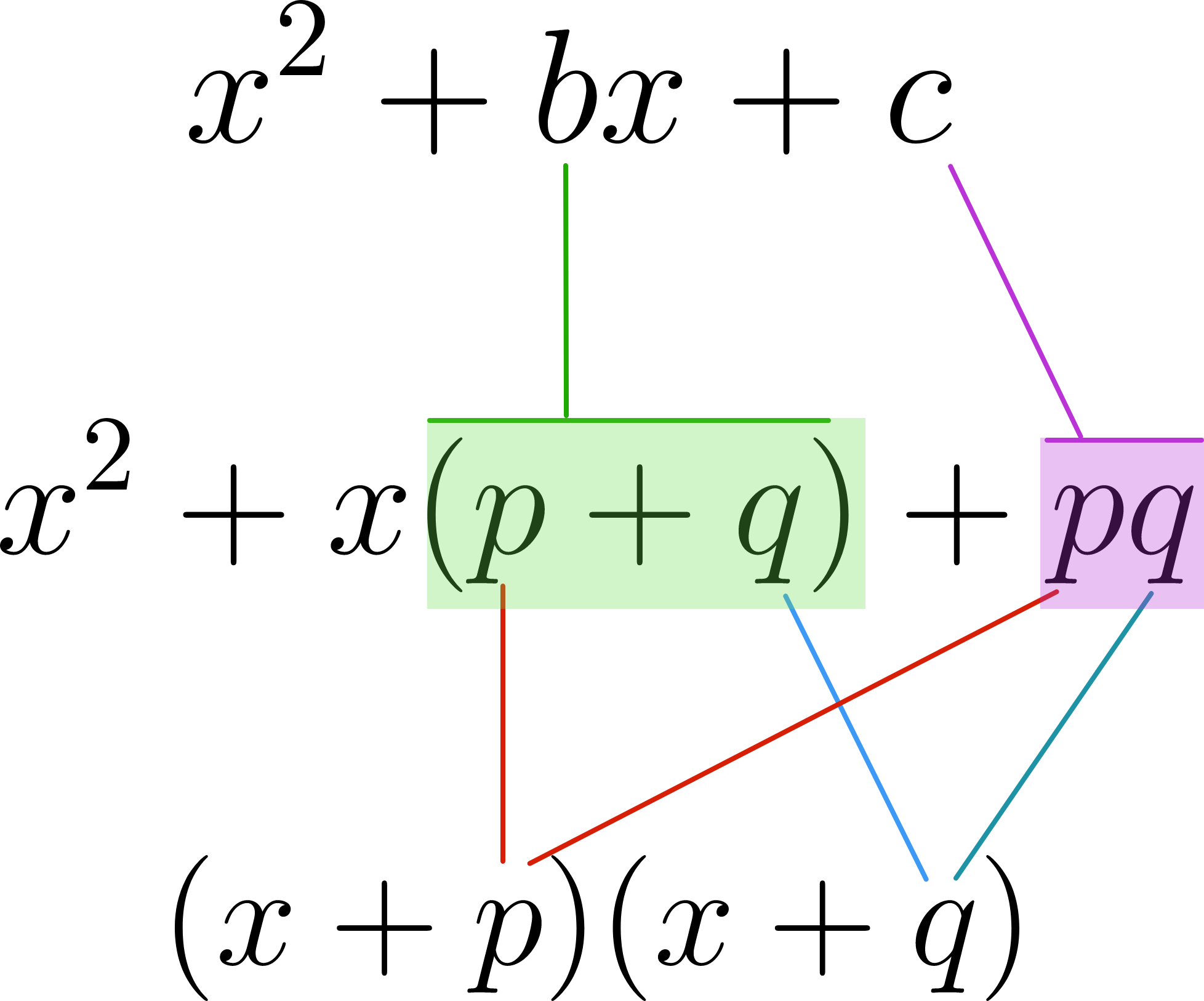### Algebra II

This course doesn't just have you explore, but it gets deep into why things are true and dives into the details of not just graphs but algebraic manipulation.

The graph of $y= x^2 - 3x - 10$ is shown below and is a parabola written in standard form. The equation can also be written in factored form as $y=(x+p)(x+q).$ Use the sliders to match the graphs and determine the values of $p$ and $q.$

What does $p + q$ equal?

# Factoring and Beyond

Let's think about factored form more generally: $f(x) = (x+p)(x+q).$ One way to multiply these is by the distributive property, getting $f(x) = x(x+p) + q(x+p).$ Continuing, $f(x) = x^2 + xp + xq + pq.$ This means, in the function $f(x) = x^2 + bx + c ,$ what does $b$ have to equal?

# Factoring and Beyond

Using the same factored form $(x+p)(x+q)$ as in the last two problems, we know that $x^2 + bx + c = x^2 + (p+q)x + pq .$ This makes it possible to factor from standard form into factored form by figuring out which values $p$ and $q$ add up to be $b$ but multiply to be $c.$But what if $x^2$ doesn't have a 1 in front? Well, we can make it have a 1 in front. (It's quite possible, even if you've done factoring in a school classroom, you've never seen this method you're about to see!)

# Factoring and Beyond

$f(x)= 7x^2 + 12x -4$ We'd like to write the function above in factored form. As this is just an introduction, we won't linger on the details, but if you multiply the original function by $\frac{1}{7}$ and $7$ (which is the same as multiplying by 1, so it's allowed), you can get \begin{aligned} f(x) &=(7x^2+12x-4 ) \\ &= \frac{1}{7}(7)(7x^2 + 12x -4) \\ &= \frac{1}{7} \big((7x)^2 + 12(7x) - 28\big). \end{aligned} Which function is equivalent?

# Factoring and Beyond

You may have come across already, given $f(x) = ax^2 + bx + c ,$ that $x = \frac{-b \pm \sqrt{b^2 - 4ac}}{2a}$ is the quadratic formula that gives both solutions to when the function equals zero $\big($and one of the factored forms, if we write the answers $k$ and $j$ as $(x-k)(x-j)\big).$

This course derives the entire formula from scratch.

For this introduction, just consider a side question: Which equation in the answer choices has no real number solutions?

Hint: There's a square root in the quadratic formula. What happens when the value inside is less than 0?

# Factoring and Beyond

Here's one last challenge; this is meant to give you a feel of what it's like to combine knowledge of factoring and graphing to attack deep problems.

When $a = 5,$ the graph of the function $f(x) = \frac{x^2-7x-60}{x+a}$ can be described as "a line with a hole in it." What's another value of $a$ that has the same effect?

# Factoring and Beyond

Here's the same graph from the previous problem, with the slider range expanded so you can see what happens at the solution.

Many more visualizations and puzzles await in the course - Function Fundamentals will start things off by exploring the structure of functions and explaining why generalizing to functions is so powerful.

×